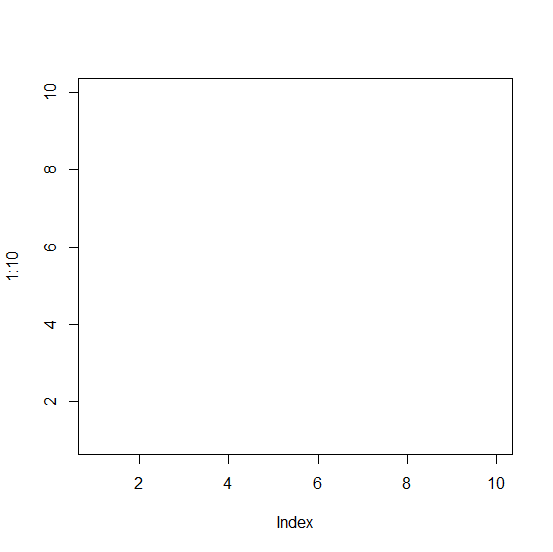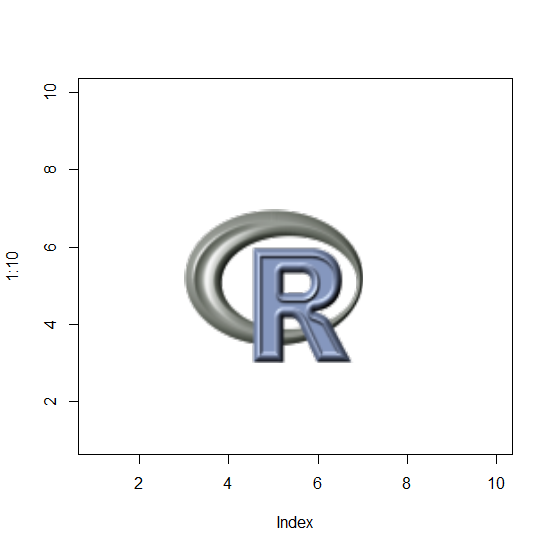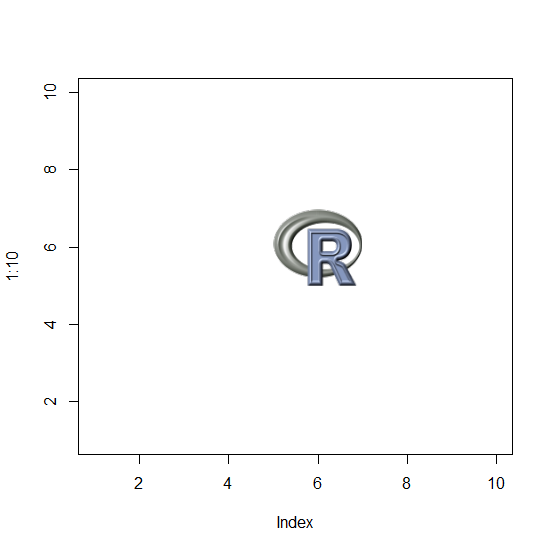# How to add a picture to plot in base R?

To add a picture to a plot in base R, we first need to read the picture in the appropriate format and then rasterImage function can be used. The most commonly used format for picture in R is PNG. A picture in PNG format can be added to a plot by supplying the values in the plot where we want to add the picture.

## Example

> library(png)

> Picture<-readPNG(system.file("img","Rlogo.png",package="png"))
Creating a blank plot:
> plot(1:10,ty="n")

## Output:Adding the picture in png file to the above plot:

## Example

> rasterImage(Picture,3,3,7,7)

## Output:## Example

> plot(1:10,ty="n")
> rasterImage(Picture,5,5,7,7)

## Output: